The Measurers:
a Flemish Image of Mathematics in the Sixteenth Century

List of Figures

Introduction: figures 1 to 3
The Mathematicians: figures 4 to 15
The Measurers: figures 16 to 31
The Collectors: figures 32 to 48

The Measurers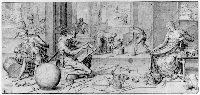Figure 16

Drawing, Les Mesures
Attributed to the school of Frans Floris.

By kind permission of the Ecole nationale supérieure des Beaux-Arts, Paris.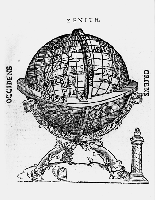Figure 17

Geographical Globe

From Peter Apian and Gemma Frisius, Cosmographia, sive descriptio universi orbis (Antwerp, 1584) (catalogue no. 2).

Note the quadrant, the pillar sundial, and tiny dipych sundial indicating the meridian.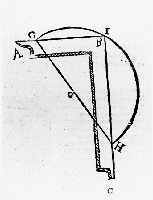Figure 18

The square used to illustrate one of the properties of a semicircle.

From Adriaan Metius, Arithmeticć et geometrić practica (1611).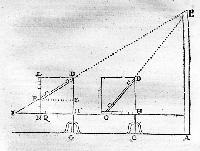Figure 19

The use of the geometrical quadrant.

From Adriaan Metius, Arithmeticć libri duo: et geometrić libri VI (Leiden, 1640).

The linear division of the sides of the square means that the angles required are taken as ratios instead of in degrees; this allows the convenient conversion of, for example, lengths measured on the ground to inaccessible heights.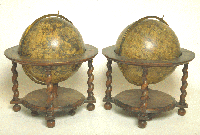Figure 20

Pair of Blaeu globes.

See catalogue no. 15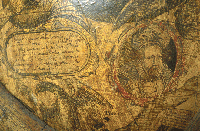Figure 21

Detail from the celestial globe showing a portrait of Tycho Brahe.

See catalogue no. 15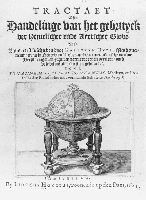Figure 22

Title-page of the Dutch edition of Hues's account of the globes, illustrating a celestial globe by Hondius.

See catalogue no. 22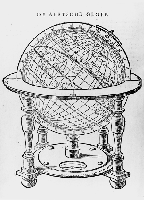Figure 23

The terrestrial globe in the Hondius edition of Hues's work.

See catalogue no. 22Figure 24

Introductory page to the first section of Blaeu's book, 'following the improper hypothesis of Ptolemy, which holds that the earth is stationary'.

See catalogue no. 23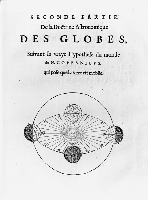Figure 25

Introductory page to the second section of Blaeu's book, 'according to the true hypothesis of the world of N. Copernicus, which proposes the motion of the earth'.

See catalogue no. 23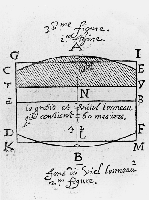Figure 26

An illustration of the use of Coignet's sector.

Coignet's sector is described in the manuscript illustrated in figure 7 and figure 8 and is used to perform calculations relating to volumes of wine in barrels.

Michel Connette [Coignet], La géometrie (Paris, 1626).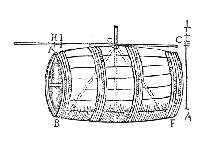Figure 27

Gauging a barrel.

From Adriaan Metius, Arithmeticć libri duo: et geometrić libri VI (Leiden, 1640).
The same woodcut appears in his Arithmeticć et geometrić practica (1611).Figure 28

Holland circle

See catalogue no. 32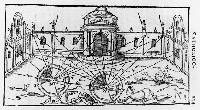Figure 29

The use of the shadow square or geometrical quadrant on an astrolabe, simple theodolite or Holland circle.

The shadow square is used to take bearings from either end of a measured baseline MN, and then to transfer these measurements on to a plan drawn to scale (see figure 30).
Gemma Frisius, De astrolabo catholico (Antwerp, 1556) (catalogue no. 3).Figure 30

The use of the shadow square or geometrical quadrant on an astrolabe, simple theodolite or Holland circle.

Bearings taken with the shadow square (see figure 29) after transfer on to a plan drawn to scale.
Gemma Frisius, De astrolabo catholico (Antwerp, 1556) (catalogue no. 3).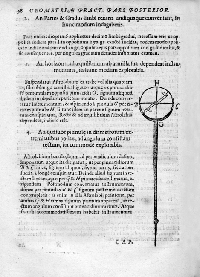Figure 31

A simple theodolite on a staff.

Illustrated in Adriaan Metius, Arithmeticć et geometrić practica (1611).

Introduction: figures 1 to 3
The Mathematicians: figures 4 to 15
The Collectors: figures 32 to 48
Contents
(c) Museum of the History of Science, Broad Street, Oxford, England.